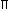1.
 If the perimeter of a right-angled isosceles triangle is 4 2 +4m, then the Hypotenuse is
• A.
8 m
• C.
4 m
• B.
6 m
• D.
None of these
• Report
Explanation :
 Perimeter of a right-angled isosceles triangle = ( 2 + 1) x hypotenuse
4 2  + 4 = ( 2  + 1) x hypotenuse
hypotenuse = 4 m
Report
 Name Email
2.
If the radius of a circle is reduced by 40%, its circumference is reduced by
• A.
60%
• C.
35%
• B.
40%
• D.
45%
• Report
Explanation :
Reduced radius = r - 0.4r = 0.6r
Percentage reduction in circumference =
 2r - 2(0.6) r
 2r
x 100 = 40%
Report
 Name Email
3.
The base of a triangular field is three times its height. If the cost of cultivating the field at Rs. 36.72 per hectare is Rs. 495.72, find its base and height.
• A.
950 m, 350 m
• C.
900 m, 300 m
• B.
800 m, 500 m
• D.
None of these
• Report
Explanation :
Area of field =
 495.72 36.72
x 10000
 = 1,35,000 m2
Let the height be x
Then, base = 3x
We have,
 1 2
x x x 3x = 1,35,000
x2 = 90000 or x = 300
Height = 300 m and base = 3 x 300 = 900 m
Report
 Name Email
4.
The adjacent sides of a parallelogram are 8 m and 5 m. The distance between the longer sides is 4 m. The distance between the shorter sides is
• A.
4.6 m
• C.
8.6 m
• B.
6.4 m
• D.
None of these
• Report
Explanation :
Area of the parallelogram = 8 x 4 = 32 m2
Distance between the shorter sides =
 32 5
= 6.4 m
Report
 Name Email
5.
A rectangular farm has to be fenced on one long side, one short side and the diagonal. If the cost of fencing is Rs. 10 per m, the area of farm is 1200 m2 and the short side is 30 m long, how much would the job cost?
• A.
Rs. 700
• C.
Rs. 1400
• B.
Rs. 1200
• D.
Rs. 1500